## 每日灵签上线啦！

You can send an email to my mailbox: flamelswift@gmail.com

Hint: You can get 40% discount by ordering 30 qians at one time!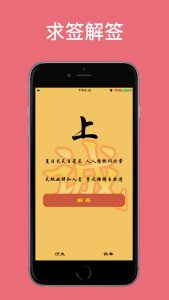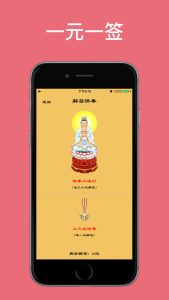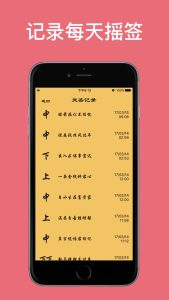## Xcode8中无法设定extension图标的解决办法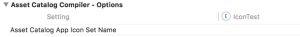## swift的计算属性

### get

get方法在这两个方法中是必须的，意即如果要声明计算属性，则必须存在get方法，get方法的作用是在开发者用dot文法获取该变量的值的时候，返回一个固定的或经过计算的值。

### set

set方法是一个可选方法，其会传入一个与所属变量类型相同的参数，这个参数的值即使用中被赋予的值，在set文法中，可以利用获取的参数的值，去计算并设定其他变量的值。如果要使用set方法，则必须包含get方法。

```struct Point {
var x = 0.0, y = 0.0
}
struct Size {
var width = 0.0, height = 0.0
}
struct Rect {
var origin = Point()
var size = Size()
var center: Point {
get {
let centerX = origin.x + (size.width / 2)
let centerY = origin.y + (size.height / 2)
return Point(x: centerX, y: centerY)
}
set(newCenter) {
origin.x = newCenter.x - (size.width / 2)
origin.y = newCenter.y - (size.height / 2)
}
}
}
var square = Rect(origin: Point(x: 0.0, y: 0.0),
size: Size(width: 10.0, height: 10.0))
let initialSquareCenter = square.center
square.center = Point(x: 15.0, y: 15.0)
print("square.origin is now at (\(square.origin.x), \(square.origin.y))")
// Prints "square.origin is now at (10.0, 10.0)"
```

#### 2. set可以忽略参数声明而直接使用newValue的默认值，如上面的例子可以被改写成这样

```set {
origin.x = newValue.x - (size.width / 2)
origin.y = newValue.y - (size.height / 2)
}
```

#### 3. 如果要声明计算属性则必须声明get方法，只声明get方法的计算属性成为只读计算属性，将来的使用中该属性无法被赋值而只能获取其在get方法中返回的值。同时，只读计算属性可以简化写法，不用再按标准方式声明get方法。例如：

```struct Cuboid {
var width = 0.0, height = 0.0, depth = 0.0
var volume: Double {
return width * height * depth
}
}
let fourByFiveByTwo = Cuboid(width: 4.0, height: 5.0, depth: 2.0)
print("the volume of fourByFiveByTwo is \(fourByFiveByTwo.volume)")
// Prints "the volume of fourByFiveByTwo is 40.0"
```

## 属性观察者

willSet方法会在新值被储存前调用，官方文档中使用了“just before”这个词，可能意思就是这个方法调用完了该变量就会立刻存储新值吧。同时willSet方法接收的参数是即将被存储的新值，即newValue，如果希望简化方法的话可以不书写参数而直接用dot文法调用该变量。

```class StepCounter {
var totalSteps: Int = 0 {
willSet(newTotalSteps) {
print("About to set totalSteps to \(newTotalSteps)")
}
didSet {
if totalSteps > oldValue {
}
}
}
}
let stepCounter = StepCounter()
stepCounter.totalSteps = 200
// About to set totalSteps to 200
stepCounter.totalSteps = 360
// About to set totalSteps to 360
stepCounter.totalSteps = 896
// About to set totalSteps to 896
```

## 1.如何修改Tabbar item的selected color

`self.tabBarController?.tabBar.tintColor = UIColor.blueColor()`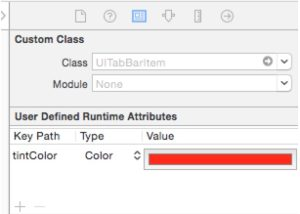## 2.判断字符串之间关系

```var myString = "This is a string test"
if myString.rangeOfString("string") {
print("exists")
}```

## 6.如何添加storyboard辅助线

storyboard中，双击某个view，再按shift+command+ -会添加横向的辅助线，shift+command+ | 会添加纵向的辅助线。删除辅助线的方法就是点击辅助线后快速移出view到旁边的空白处

## 8.将Int等其他类型的变量变为字符串输出

“\(times[row])”,这样就可以输出原本为int数组的times中得值了。

## 9.读取与写入plist的方法

```var partArray : NSArray?   //声明存储plist文件数据的数组
let ban = NSBundle.mainBundle()//获取mainbundle的值，这里面保存了plist文件的地址（注意plist文件必须创建在主文件路径下才可以）
let plistPath = ban.pathForResource("myNumber" , ofType : "plist”)//连接plist文件
let partFromP = NSMutableDictionary(contentsOfFile: plistPath!)//将plist文件存入一个数组
partArray = partFromP?.objectForKey("肩部") as? NSArray  //通过key值寻找值```

IOS设备写入plist文件时，不能使用mainbundle来寻找文件路径，这样只会导致写入失败，以下是正确地寻找文件路径的方法：

```let paths = NSSearchPathForDirectoriesInDomains(.DocumentDirectory, .UserDomainMask, true) as NSArray
let documentsDirectory = paths.objectAtIndex(0) as! NSString
let path = documentsDirectory.stringByAppendingPathComponent("GameData.plist")
//以上三行获取文件地址就不细说了，下面这行是将文件中的值保存到一个NSDictionary中
let mydict = NSDictionary(contentsOfFile: path)
//获取用户数组
var partArray : NSArray = mydict?.objectForKey("users") as! NSArray
//需要新加的数据
var object = [NSDictionary]()
object.append(["part" : "上臂" , "move" : "肩部挺举" ,"group":"3","times":"15"])
//返回一个新的数组